• FAQ
• Contact/ Homework Answers / Accounting / 41.The cost formula for the maintenance department of the Eifel
Not my Question
Flag Content

# Question

41.The cost formula for the maintenance department of the Eifel Co. is \$6,500 per month plus \$3.50 per machine hour used by the production department.

a. Calculate the maintenance cost that would be budgeted for the month of May in which 5,700 machine hours are planned to be used.
b. Prepare an appropriate performance report for the maintenance department assuming that 5,860 machine hours were actually used in the month of May, and the total maintenance cost incurred was \$28,010.

42.The standards for one case of Saycheles are: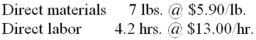During the week ended April 15, the following activity took place:

• 14,500 lbs. of raw material were purchased for inventory at a total cost of \$81,200;
• 1,800 cases of finished product were produced;
• 13,680 lbs. of raw material were used;
• 7,380 labor hours were worked at an average rate of \$13.40 per hour.

Calculate each of the following variances:

(a.) Raw materials purchase price variance.
(b.) Raw materials usage variance.
(c.) Direct labor rate variance.
(d.) Direct labor efficiency variance.
(e.) Explain the factors that most likely caused the variances computed above.

43.The standards for one carton of Wonder Wax are: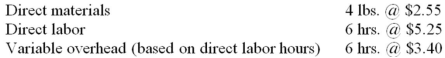During the week ended December 4, the following activity took place:

• 4,600 lbs. of raw material were purchased for inventory at a cost of \$2.63 per pound;
• 1,050 cartons of finished product were produced;
• 4,225 lbs. of raw material were used;
• 6,140 labor hours were worked at a total cost of \$33,463;
• \$20,569 actual variable overhead costs were incurred.

Calculate each of the following variances:

(a.) Price variance for raw materials purchased.
(b.) Raw materials usage variance.
(c.) Direct labor rate variance.
(d.) Direct labor efficiency variance.

44.The standards for one carton of Flavor Rite are: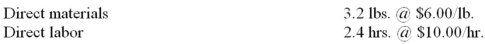During the week ended May 17, the following activity took place:

• 4,800 lbs. of raw material were purchased for inventory at a cost of \$5.60 per pound;
• 1,200 cartons of finished product were produced;
• 4,020 lbs. of raw material were used;
• 3,110 labor hours were worked at a total cost of \$26,435.

Calculate each of the following variances:

(a.) Raw materials purchase price variance.
(b.) Raw materials usage variance.
(c.) Direct labor rate variance.
(d.) Direct labor efficiency variance.
(e.) Explain the factors that most likely caused the variances computed above.

45.The standards for one case of Peardrax are: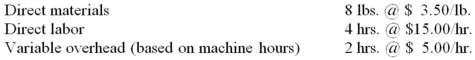During the week ended June 7, the following activity took place:

• 5,740 machine hours were worked;
• 22,600 lbs. of raw material were purchased for inventory at a total cost of \$83,620;
• 2,700 cases of finished product were produced;
• 21,330 lbs. of raw material were used;
• 10,650 labor hours were worked at an average rate of \$15.20 per hour;
• \$27,552 actual variable overhead costs were incurred.

Calculate each of the following variances:

(a.) Price variance for raw materials purchased.
(b.) Raw materials usage variance.
(c.) Direct labor rate variance.
(d.) Direct labor efficiency variance.

46.The Diamond Manufacturing Company uses a standard cost system for and applies overhead based on machine hours. The following information is available for June: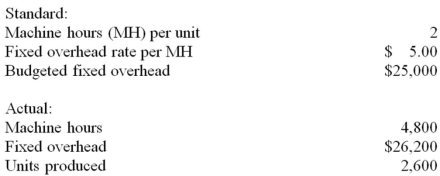Calculate the fixed overhead budget variance and volume variance for the month of June.

47.GrandGlaze Co. is divided into three segments and is interested in preparing a segmented income statement in order to better understand the operating performance of each segment. Fixed expenses in each division currently include an allocation of general corporate expenses equal to 20% of the division's sales.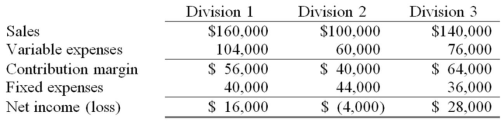(a.) Complete the following segmented income statement for GrandGlaze Co.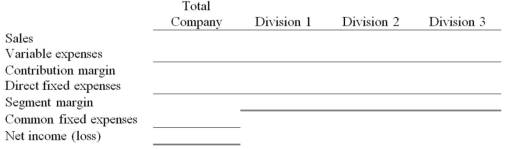48.ABC, Inc., is segmented into three divisions and the company is concerned about the performance of Division Y. During your analysis of Division Y, you learn that \$42,000 of the fixed expenses relate to general corporate expenses and had been allocated equally between the three divisions.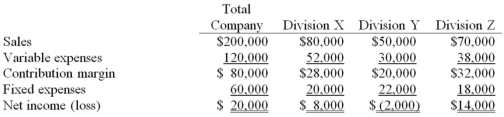(a.) Calculate what the company's net income would be if Division Y were closed down.
(b.) Revise the income statement presented above into a more useful segmented income statement.

49.The Eastern Division of XYZ, Inc., has operating income of \$32,000 on sales revenue of \$320,000. Divisional operating assets are \$160,000 and management of XYZ has determined that a minimum return of 10% should be expected from all investments.

(a.) Using the DuPont model, calculate the Eastern Division's margin, turnover, and ROI.
(b.) Calculate the Eastern Division's residual income.

50.Selected information about American Industries is presented below. American has three operating divisions and requires a 12% return on all investments: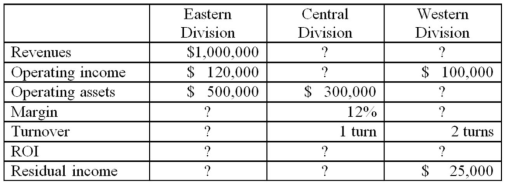Calculate the missing amounts for each division.

15-1

## Solution 5 (1 Ratings )

Solved
Accounting 3 Months Ago 528 Views